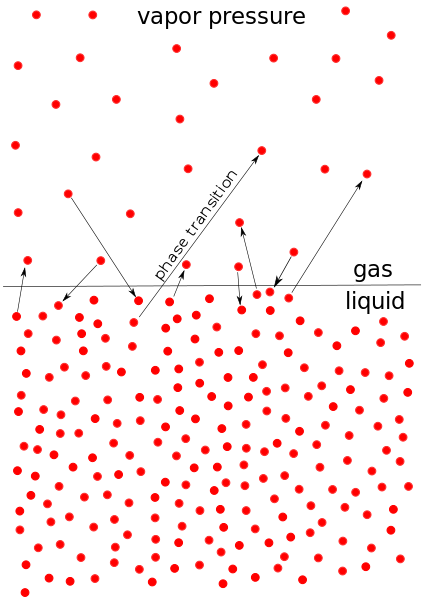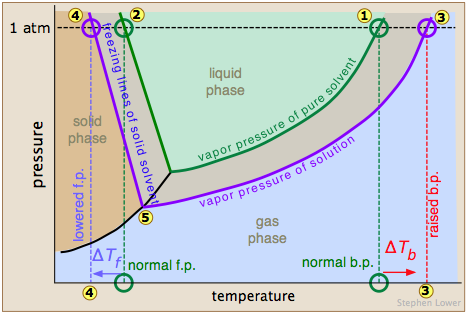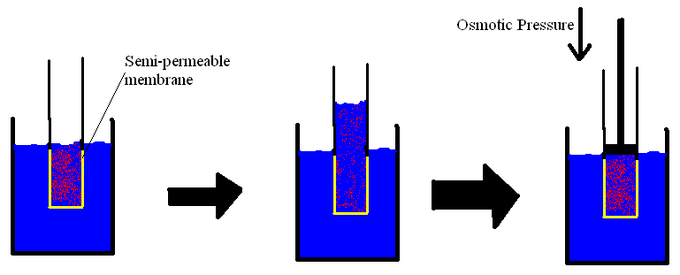## Vapor Pressure of Nonelectrolyte Solutions

The vapor pressure of a solution is directly influenced by the number of solute molecules present in a given amount of solvent.

### Learning Objectives

Calculate the vapor pressure of a nonelectrolyte solution using Raoult’s law

### Key Takeaways

#### Key Points

• The vapor pressure of a system is a measure of the molecules ‘ tendency to escape into the gas phase.
• Vapor pressure is a colligative property, meaning that the amount it is increased or decreased is directly related to the amount of solute present in the system.
• Raoult’s law states that the partial vapor pressure of each component of an ideal mixture of liquids is equal to the vapor pressure of the pure component multiplied by its mole fraction in the mixture

#### Key Terms

• Raoult’s law:
• raoult’s law: States that the partial vapor pressure of each component of an ideal mixture of liquids is equal to the vapor pressure of the pure component multiplied by its mole fraction in the mixture
• colligative property: A property of solutions that is directed by the ratio of solute to solvent, regardless of the identity of the solute.
• vapor pressure: The pressure that a vapor exerts, or its partial pressure if it is mixed with other gases.

### Colligative Properties: Vapor Pressure

Vapor pressure is a measure of the tendency of a material to escape into the environment via gas. A substance that evaporates quickly has high vapor pressure and is referred to as a volatile substance. When a solute is added to a solvent, the vapor pressure decreases.Vapor pressure: Vapor pressure measures the particle transition from the liquid phase to the gas phase and vice versa.

### Lowering Vapor Pressure

The vapor pressure of a solvent is lowered by the addition of a non-volatile solute to form a solution. By definition, a non-volatile substance does not evaporate. This decrease in vapor pressure can be explained by using the entropy differences of the liquid and gas phases along with the position of dissolved particles after the addition of the solute.

### Entropy

When molecules transition from the liquid phase to the gas phase, entropy of the system increases. Entropy of the gaseous state is greater than the entropy of the liquid state because the gaseous molecules occupy a larger volume.

If the liquid solvent becomes “diluted” with solute, the entropy of the liquid state increases. Therefore, even though the gaseous state has a higher entropy, the difference in entropy between the two systems decreases. The decrease in entropy difference lowers the vapor pressure.

### Solute Particle Location

In a pure solvent, all of the liquid molecules on the surface can evaporate and transition to the gaseous phase. In a solution, some spots on the surface are occupied by solute molecules, so there is less space occupied by solvent molecules. Overall, less solvent will transition to the gaseous phase, resulting in a decrease in vapor pressure.

### Equilibrium Vapor Pressure Given by Raoult’s Law

Raoult’s law states that the vapor pressure of an ideal solution is dependent on the vapor pressure of the pure solvent and the mole fraction of the component present in the solution. For an ideal solution, equilibrium vapor pressure is given by Raoult’s law:

$\text{p} = \text{p}^{\star}_{\rm \text{A}} \text{x}_{\rm \text{A}}$

In this equation, $\text{p}^{\star}_{\rm \text{A}}$ is the vapor pressure of the pure solvent and $\text{x}_{\rm \text{A}}$ is the mole fraction of the solvent. This law allows us to calculate the vapor pressure of a given solution, and shows the influence of all of the molecules in the solution.

### Example

Calculate the vapor pressure of a solution consisting of 3 moles of a nonvolatile solute and 15 moles of water at 25 oC, given that the vapor pressure of pure water at 25 oC is 23.8 torr.

First, calculate the mole fraction of the solvent:

$\text{x}_{\rm \text{A}} = \frac{\text{moles}\ \text{solvent}}{\text{total}\ \text{moles}}$

$\text{x}_{\rm \text{A}} = \frac{15}{18} = 0.83$

Second, substitute into Raoult’s law and solve:

$\text{p} = \text{p}^{\star}_{\rm \text{A}} \text{x}_{\rm \text{A}}$

$\text{p} = 23.8\ \text{torr} \times 0.83$

$\text{p} = 19.8\ \text{torr}$

### Freezing Point Depression

Freezing point depression is a colligative property observed in solutions, brought on by the introduction of solute molecules to a solvent.

### Learning Objectives

Discuss the effects of a solute on the freezing point of a solvent

### Key Takeaways

#### Key Points

• The freezing point depression can be calculated using the pure solvent freezing point and the molality of the solution.
• At the freezing point, the vapor pressure of both the solid and liquid form of a compound must be equal.
• The freezing point of a substance is the temperature at which the solid and liquid forms are in equilibrium.
• To reattain equilibrium, the freezing point of the solute and solvent mixture is lowered relative to the original pure solvent.

#### Key Terms

• freezing point depression: Adding a solute to a solvent decreases the temperature at which the liquid solvent becomes a solid.
• vapor pressure: The pressure that a vapor exerts, or the partial pressure if it is mixed with other gases.
• van ‘t Hoff factor: A measure of the effect of a solute on colligative properties.
• freezing point: The temperature at which a liquid freezes, and the solid and liquid phases are in equilibrium; normally the same as the melting point.

Freezing point depression is the phenomena that describes why adding a solute to a solvent results in the lowering of the freezing point of the solvent. When a substance starts to freeze, the molecules slow down due to the decreases in temperature, and the intermolecular forces start to take over. The molecules will then arrange themselves in a pattern, and thus turn into a solid. For example, as water is cooled to the freezing point, its molecules become slower and hydrogen bonds begin to “stick” more, eventually creating a solid. If salt is added to the water, the Na+ and Cl ions attract to the water molecules and interfere with the formation of the large network solid known as ice. In order to achieve a solid, the solution must be cooled to an even lower temperature.

The freezing point depression can also be explained in terms of vapor pressure. Adding solute to a solvent will essentially dilute the solvent molecules, and according to Raoult’s law, this leads to a decrease in vapor pressure. Considering the fact that the vapor pressure of the solid and liquid forms must be the same at freezing point, because otherwise the system would not be at equilibrium, the lowering of the vapor pressure leads to the lowering of the temperature at which the vapor pressures of the liquid and frozen forms of the solution will be equal.Effect of solutes on physical properties: A triple phase diagram which shows the pressure and temperature of the normal boiling and freezing points of a solvent (green lines) and the boiling and freezing points of a solution (purple lines). Notice that at 1 atm of pressure, the freezing point has been lowered (represented by numbers 2 and 4).

The freezing point depression can be calculated by the formula:

$\Delta \text{T}_\text{f} = \text{i}\times \text{K}_\text{f} \times \text{molality}$

In this equation, $\Delta \text{T}_\text{f}$ is the freezing point depression, Kf is the freezing point depression constant, and i is the van ‘t Hoff factor. The freezing point depression constant changes depending on the solvent, and the van ‘t Hoff factor accounts for the number of particles that a dissolving solute creates in solution.

### Example

What is the freezing point of an aqueous solution when enough NaCl has been added to create a 0.25 m solution? The Kf value for water is 1.858 oC/m.

To solve this, you must remember that NaCl breaks into two ions, Na+ and Cl, when it dissolves in water. In simplest terms, this means it has an “i” factor of 2.

$\Delta \text{T}_\text{f} = \text{i}\times \text{K}_\text{f} \times \text{molality}$

$\Delta \text{T}_\text{f} = 2\times 1.86 \frac{^\text{oC}}{\text{m}} \times 0.25\ \text{m}$

$\Delta \text{T}_\text{f} = 0.93^\text{oC}$

This might seem like the end of the problem, but it is not. The value of 0.93 oC is the change in the freezing point. The new freezing point of water, which is normally 0 oC, is equal to: 0 – 0.93 = -0.93 oC.

### Boiling Point Elevation

The boiling point of a solvent is elevated in the presence of solutes.

### Learning Objectives

Discuss the effects of a solute on the boiling point of a solvent

### Key Takeaways

#### Key Points

• One of the colligative properties of a solution is boiling point elevation.
• The amount that the boiling point increases in the presence of solute can be calculated by using the boiling point elevation constant and the molality of the solution.
• The addition of solute dilutes the solvent molecules and makes it harder for them to escape into the gaseous phase.

#### Key Terms

• boiling point: The temperature at which a liquid boils, with the vapor pressure equal to the given external pressure.
• boiling point elevation: The temperature at which a substance’s vapor pressure equals the external pressure increases when another compound is added.
• colligative property: A property of solutions that is directed by the ratio of solute to solvent, regardless of the identity of the solute.

### Colligative Properties and Boiling Point Elevation

There is one category of properties that can only be applied to solutions; these are known as colligative properties. Properties can be considered colligative only if they are dependent on the amount of solute present in the solution, disregarding the identity of the solute itself.

The boiling point of a solvent will increase when a solute is dissolved in it. This is referred to as boiling point elevation. The elevation of the boiling point is directly dependent on the amount of solute present in the solution, but it is not based on the identity of the solute, so it is considered a colligative property.

### The Relationship Between Boiling Point Elevation and Vapor Pressure

Boiling point elevation can be explained in terms of vapor pressure. Vapor pressure is defined as the pressure exerted by a vapor in thermodynamic equilibrium with its condensed phases at a given temperature. In layman’s terms, it is simply a measure of the tendency of the solution molecules to escape by entering the gas phase. A liquid boils when its vapor pressure is equal to the air pressure.Boiling point: The boiling point of a pure liquid. When the vapor pressure of the liquid matches the atmospheric pressure, the liquid will boil.

### Boiling Point Elevation

A solvent’s vapor pressure will lower when a solute is added. This happens because of the displacement of solvent molecules by the solute. This means that some of the of solvent molecules at the surface of the liquid are replaced by the solute; it can occur in both electrolytic and non-electrolytic solutions. The lower number of solvent molecules at the surface means that fewer will evaporate, and thus the vapor pressure is lowered. For the vapor pressure to equal the atmospheric pressure, a higher temperature is required, and a higher boiling point is observed.

### Calculating Boiling Point Elevation

The extent of the boiling point elevation can be calculated. It is directly proportional to the molal concentration of the solution. The amount the boiling point is elevated is determined using the equation:

$\Delta \text{T}_\text{b} = \text{i} \times \text{K}_\text{b} \times \text{m}$

In this equation, $\Delta \text{T}_\text{b}$ is the boiling point elevation, $\text{K}_\text{b}$ is the boiling point elevation constant, and m is the molality of the solution. The “i” factor of the equation also factors in any dissociation that the solute may undergo; since boiling point elevation is a colligative property, the number of ions present in an electrolyte affects the elevation.

### Example

Calculate the boiling point of an aqueous solution where enough NaCl is added to make a 0.37 molal solution. The Kb for water is 0.512 $\frac {^\text{oC}}{\text{m}}$.

$\Delta \text{T}_\text{b} = \text{i} \times \text{K}_\text{b} \times \text{m}$

$\Delta \text{T}_\text{b} = 2 \times 0.512 \frac{^\text{oC}}{\text{m}} \times 0.37\ \text{m}$

$\Delta \text{T}_\text{b} = 0.38^\text{oC}$

Water normally boils at 100 oC, so the new boiling point of the solution would be 100.38 oC.

### Osmotic Pressure

Osmotic pressure is the pressure needed to nullify the effects of osmosis and is directly influenced by the amount of solute in the system.

### Learning Objectives

Discuss the effects of a solute on the osmotic pressure of a solution

### Key Takeaways

#### Key Points

• Osmosis is defined as the flow of water/ solvent molecules through a semipermeable membrane from a region of low to high solute concentration, until equilibrium is established.
• To counter osmotic flow, some pressure must be applied to the solution in order to prevent pure solvent from going through the semipermeable membrane separating the two liquids; this is known as the osmotic pressure.
• The osmotic pressure is the pressure required to counter, not sustain, osmosis.
• The osmotic pressure can be approximated by using the following formula: $\Pi = \text{i} \text{M} \text{R} \text{T}$.

#### Key Terms

• osmotic pressure: The hydrostatic pressure exerted by a solution across a semipermeable membrane from a pure solvent; the pressure needed to counteract osmosis.
• semipermeable membrane: One that will allow certain molecules or ions to pass through it by diffusion.
• osmosis: The net movement of solvent molecules from a region of high solvent potential to a region of lower solvent potential through a partially permeable membrane.
• ideal solution: A solution with thermodynamic properties analogous to those of a mixture of ideal gases.

A solution is defined as a homogeneous mixture of both a solute and solvent. Solutions generally have different properties than the solvent and solute molecules that compose them. Some special properties of solutions are dependent solely on the amount of dissolved solute molecules, regardless of what that solute is; these properties are known as colligative properties.

Osmosis is defined as the net flow or movement of solvent molecules through a semipermeable membrane through which solute molecules cannot pass. If a solution consisting of both solute and solvent molecules is placed on one side of a membrane and pure solvent is placed on the other side, there is a net flow of solvent into the solution side of the membrane.

Imagine osmosis taking place in an upright U-tube. The height of the solution will continue to increase due to a net flow of solvent until the added pressure of the height will cause the flow of solution to stop. The height difference between the two sides can be be converted into pressure to find the osmotic pressure exerted on the solution by the pure solvent.U-Tube showing osmotic pressure: On the left side of the U-tube is an aqueous solution, and on the right side is pure water. The pure water is trying to dilute the solution by travelling through the semipermeable membrane. Eventually the added weight of the extra water on the left causes enough pressure to stop osmosis.

Osmotic pressure is the pressure that needs to be applied to a solution to prevent the inward flow of water across a semipermeable membrane. Osmotic pressure can also be explained as the pressure necessary to nullify osmosis. One way to stop osmosis is to increase the hydrostatic pressure on the solution side of the membrane; this ultimately squeezes the solvent molecules closer together, increasing their “escaping tendency.” The escaping tendency of the solution can be raised until it eventually equals that of the molecules in the pure solvent; at this point, osmosis will cease. The osmotic pressure is the pressure required to achieve osmotic equilibrium.Osmotic pressure: Osmotic pressure is the pressure required to stop osmosis.

The osmotic pressure (II) of an ideal solution can be approximated by the Morse equation:

$\Pi = \text{i} \text{M} \text{R} \text{T}$

Here, i is the van ‘t Hoff factor, M is the molarity of the solution, R is the gas constant, and T is the absolute temperature in Kelvin. We can see from this equation that the amount of solute present in the solution will directly affect the osmotic pressure of the system.

### Example

What is the osmotic pressure of a 1.35 M solution of NaCl at 25 oC?

First, fill in all of the necessary information, and then solve:

i = 2 (NaCl breaks into two particles)

M = 1.35 $\frac{\text{moles}}{\text{L}}$

R = 0.0821 $\frac{\text{L}\times \text{atm}}{\text{K} \times \text{mol}}$$\frac{\text{L}\times \text{atm}}{\text{K} \times \text{mol}}$

T = 25 oC + 273 = 298 K

$\Pi = 2 \times 1.35 \times 0.0821 \times 298$

$\Pi = 66.1\ \text{atm}$